American Journal of Civil Engineering
Volume 4, Issue 1, January 2016, Pages: 24-39

Risk and Uncertainty Assessment Model in Construction Projects Using Fuzzy Logic

Hesham Abd El Khalek, Remon Fayek Aziz, Hamada Mohamed Kamel*

Construction Engineering and Management, Faculty of Engineering, Alexandria University, Alexandria, Egypt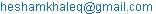(H. A. El Khalek)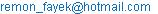(R. F. Aziz)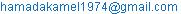(H. M. Kamel)

Hesham Abd El Khalek, Remon Fayek Aziz, Hamada Mohamed Kamel. Risk and Uncertainty Assessment Model in Construction Projects Using Fuzzy Logic. American Journal of Civil Engineering. Vol. 4, No. 1, 2016, pp. 24-39. doi: 10.11648/j.ajce.20160401.13

Abstract: In the global construction market, most construction companies are willing to undertake international projects in order to maximize their profitability by taking advantage of attractive emerging markets and minimize dependence on local market. Due to the nature of construction works, there are lots of risks and uncertainties associated with the company and project conditions. Therefore, how the profitability of the project changes with occurrence of various risk events, in other words, the sensitivity of project costs to risk events, should be estimated realistically. This paper offers a comprehensive risk assessment methodology that provides a decision support tool, which can be utilized through the bidding decisions for international construction projects introducing a risk model that facilitate this assessment procedure, prioritize these projects and evaluate risk contingency value. The risk models is developed using the analytic hierarchy process (AHP) to evaluate risk factors weights (likelihood) and FUZZY LOGIC approach to evaluate risk factors impact (Risk consequences) using software aids such as EXCEL and MATLAB software. The reliability of the developed software has been tested by applications on a real construction projects. The proposed methodology and decision support tool have been proved to be reliable for the estimation of cost overrun due to risk while giving bidding decisions in international markets. Therefore, the developed model can be used to sort projects based upon risk, which facilitate company’s decision of which project can be pursued. The developed risk model is validated, which prove its robustness in risk assessment (97%) in company level and (105%) in project level. It can also be used to sort construction projects based upon risk. The developed contingency risk model demonstrate the ability to evaluate risk contingency value by aggregating rules combining company risk index and project risk index using fuzzy logic approach and MATLAB software.

Keywords: Risk Management, International Construction, Risk Factors, Analytic Hierarchy Process (AHP), Fuzzy Logic Approach, MATLAB Software, Validation Process

1. Introduction

Risks cause cost overrun and schedule delay in many projects. The effectiveness of risk management becomes an important issue in project management . The subjective judgment could not determine the exact influence of the qualitative decision factors on the project risk. The subjective judgment could constrain or even neglect the possible strategies to improve the qualitative decision. The decision to attend to international construction projects demand a good identification and consideration of several simultaneous dimensions (e.g., maximization of project revenues, minimization and allocation of project risks, availability of finance, etc.). Hence, a multi factor decision methodology should be used to evaluate the various factors affecting the company’s analysis. [4,13,1,10].

The aforementioned decisions are complex as they are influenced by several parameters and because most parameters have a subjective, non-quantifiable, nature.

Dias (1995),  addresses the evaluation of infrastructure projects from the contractors point of view and, in doing so, elaborates a risk model that has two main objectives: 1. To provide a logical, reliable and consistent procedure that facilitates a company’s decision to engage in an infrastructure project through the analysis of different parameters, 2. To allow sensitivity analysis to be performed such that prospective companies can evaluate how different scenarios (e.g., risk mitigation strategies). [4,13,6,7].

The tool that will be described in this research represents a system is able to find the relationships among these decision factors and the effect of each factor on the total Project risk. It uses a modelling technique based on the Analytical hierarchy Process (AHP), fuzzy logic.

2. Background

Construction projects are exposed to an uncertain environment due to its enormous size (physical, manpower requirement and financial value), complexity in design technology and involvement of external factors. These uncertainties can lead to several changes in project scope during the process of project execution. Unless the changes are properly controlled, the time, cost and quality goals of the project may never be achieved .

The ability to analyse situations and to make good decisions is a very important aspect of any managerial work. The decision making process involves several tasks: planning, generating a set of alternatives, setting priorities, choosing a best policy after finding a set of alternatives, allocating resources, determining requirements, predicting outcomes, designing systems, measuring performance, insuring the insuring the stability of a system, optimizing, and resolving conflict [20,21,22,23].

The early definitions of DSS identify it as a system intended to support managerial decision makers in semi-structured decision situations. DSS were meant to be adjunct to decision makers, to extend their capabilities but not to replace their judgment . A DSS is an interactive, flexible, and adaptable Computer Based Information System (CBIS) that utilizes decision rules, models, and model base coupled with a comprehensive database [6,7,11,19]. The complexity in construction engineering often results in hesitation on the part of the decision maker in selecting specific alternatives. Fuzzy risk assessment provides a promising tool to quantify risk ratings where the risk impacts are vague and defined by subjective judgments rather than objective data. It is also a suitable technique to deal with the out of control factors: site, labour, equipment, climate, unforeseen circumstances, time dependence situations, and regulations .

Thus, fuzzy logic formulation and computation is applied in a number of engineering tasks ranging from risk assessment, to risk pricing algorithm, to construction time-cost trade-off and to whole life costs of building elements. Examples of fuzzy set theory application in construction industry are specified below.

Hyun-Ho et al., (2004) developed a risk assessment methodology for underground construction projects. The main tool of this methodology is risk analysis software. The risk analysis software is built upon an uncertainty model based on fuzzy concept. The fuzzy-based uncertainty model is designed to consider the uncertainty range that represents the degree of uncertainties involved in both (1) probabilistic parameter estimates and (2) subjective judgments. Moreover, they concluded that the proposed risk assessment methodology will provide rational and practical solutions to the insurance companies and contractors with its flexible and easy to- follow procedure and tools, and robust uncertainty modelling capability. 

Sou-Sen et al. proposed optimal construction time-cost trade-off method developed concerning the effects of both uncertain activity duration and time-cost trade-off are taken into account in this method. Fuzzy set theory is used to model the uncertainties of activity durations. The method provides an insight into the optimal balance of time and cost under different risk levels defined by decision makers .

Wang et al. (2004) developed a generic elemental whole life costing model by using the fuzzy logic model. The relationship between the context of use and the cost items is modelled by using linguistic data of experts. This model proved that fuzzy logic approach, which uses experts’ knowledge, overcomes lack of data and the uncertainty in forecasting future events. It is anticipated that this model could provide a very wide range of use in estimating whole life costs of public service buildings, such as hospital, school, etc. 

Dikmen I et al (2007) developed a model based on fuzzy rating approach is utilized to quantify cost overrun risk in international projects in the bidding stage. The step-wise procedure is developed for this approach. The step-wise procedure followed during the development of the fuzzy risk rating tool. 

Cardona and Carreño (2004) proposed fuzzy linguistic values which represent factors risk performance, such linguistic values are the same as a fuzzy set that have a membership function of the bell function. They proposed that effectiveness obtained by the defuzzification of the linguistic values is the same as a function of the Sigmoidal, so risk effectiveness is nonlinear, due to it is a complexity. 

Qammaz A (2007) introduced Structure of the International Construction project Risk (ICPRR) Software Application which was built with "Oracle Forms" . (Dias, 1995), (Salman A, 2003) and (Zayed, 2008) proposed risk models in both company and project levels based on equation (1) which represents likelihood multiplied by consequences and they use questionnaire to determine the expected risk performance of each factors and liner equation to evaluate risk effectiveness [4,27,24]. Salman. A, 2003 prove that the risk consequences drives the action as The model results is very sensitivity for any variation in worth score more than importance weight. This leads to the conclusion that the driving force in the model is the worth scores and the action may be more single based on worth score rating than importance weights , so this paper used fuzzy logic to evaluate risk performance and nonlinear Function(sigmoidal function) to evaluate risk effectiveness.

3. Study Objectives and Methodology

The objectives of current research are:

1. Identify weights of risk and uncertainty for each factor using assessment model based on analytic hierarchy process (AHP), Identify the risk performance for each factor based on developed program based on(fuzzy logic approach) using MATLAB software instead of depending on questionnaire in the previous methods

2. Identify the worth score (effectiveness) of each risk factors using nonlinear function.

3. Design flexible assessment model based on Excel sheet program to evaluate the risk using analytic hierarchy process (AHP),

4. fuzzy logic and over all Excel sheet program.

5. The results of risk analysis are used to determine the logical risk contingency for project.

Study Methodology.

This study passed through different methodology phases to achieve its objectives determining the risk index (R). Fig. 1 shows these phases and their interrelationship. They are described in detail throughout the entire paper and can be briefly listed as follows:

Stage 1: Literature Review:

Exploring previous decision support systems approaches in the field of risk evaluation. Shedding lights on the components of risk model components.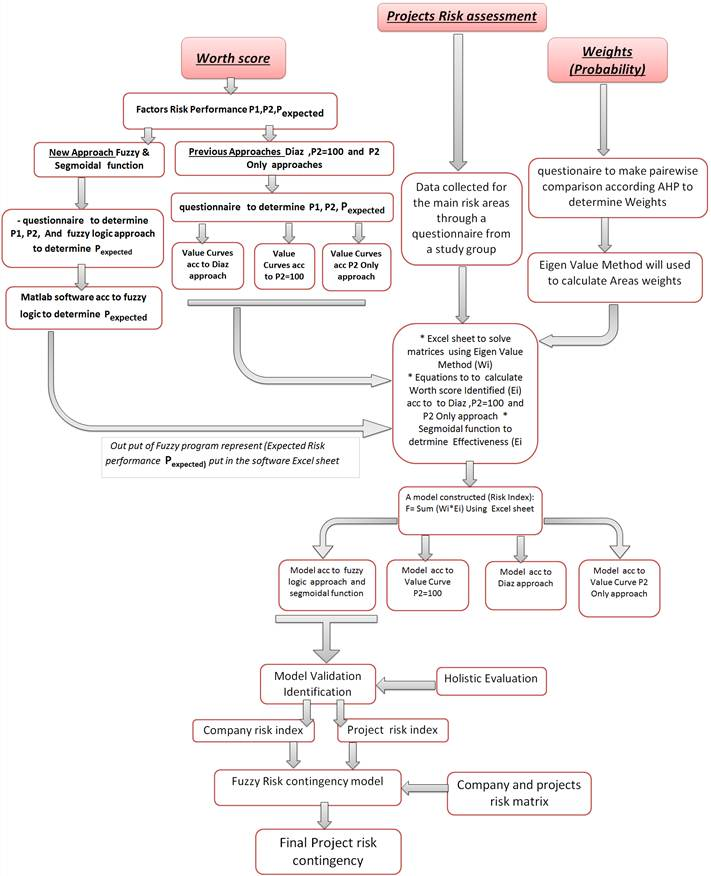Fig. 1. Study methodology flowchart.

Stage 2: Analytical study

1. Exploring model for risk evaluation in both company level and project level.

2. A two risk index (R) models in both company and project levels will be designed to assess the effect of sources of risk and uncertainty on construction project based on the equation.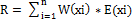Equation 1 (Dias, 1995). 

R: Risk index for a construction projects.

Wi (xi): Weight for each risk area i using Eigen value method.

Ei (xi): Effect score for each risk area (xi).

Xi: Different risk areas i

I: 1, 2, 3,............, n.

N: Number of risk areas.

3. Two models will be constructed to determine the risk index (R) and the risks areas will be divided in two levels (company level and project level). Each model consist of two parts: risk areas’ weights (W) and their worth score (E). Risk areas’ weights will be determined using the AHP; while the worth scores (Risk effectiveness) will be assessed using four approaches, Dias approach , Value curve approach according to Zayed T , New approach according to Salman M.  and Fuzzy logic approach.

Stage 3: Cases study

1. Five case studies have been employed to demonstrate the application of proposed model through questionnaire which collects information about risk sources from cross-country pipeline project and its factors from a study group.

2. Validation process will be performed to evaluate different methods by comparing their results and using four statistical evaluation methods.

Developed models through research.

There are four models were developed through research stages, table 1 illustrate the description and the objectives of each model. Hierarchy risk model in both company and project levels is displayed in fig. 2 will be used through paper to assess the projects risk.

A risk index (R) model is designed to assess the effect of sources of risk and uncertainty on a construction project from contractor (company) prospective. It provides a logical, reliable, and consistent method of evaluating potential projects, prioritizing them, and facilitating company’s decision in the promotion. The risk index (R) model based upon equation 1 characterizes the various sources of risk and uncertainty in a project and assesses their effect on such project. The R-index consists of two parts: weights of risk factors and sub-factors and their effect score. Weights of risk areas will be determined using

Table 1. Developed models which were used through study stages.

 Model No Description Objectives Model 1 Hierarchy Risk model factors Building risk model factors for both company and project level Model 2 Expected risk performance based on fuzzy logic approach Identifying Expected risk performance using MATLAB software instead of using questionnaire in the previous methods Model 3 Overall Excel sheet model Receive output results from expected performance FUZZY program, calculate each risk factor effectiveness using sigmoidal function, solving AHP matrices and calculate final project risk index Model 4 Fuzzy risk contingency model Receive output results of risk indexes for both company and project risk contingency using MATLAB software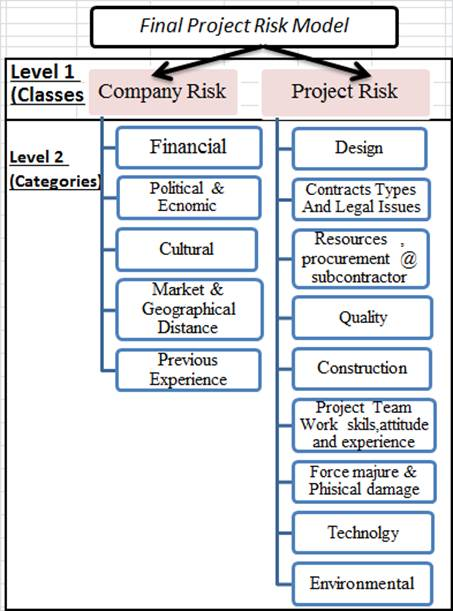Fig. 2. Risk hierarchy model in company and project Levels.

AHP developed by Saaty [20,21,22,23], however, the effect score will be assessed using utility function and fuzzy logic approaches. There are four approaches are used in developing the worth score (Impact) of the risk factors; these approaches are displayed in table 2.

1. Diaz Value Curve use two points P1, P2’s to describe the value curve. P1 is the minimum risk performance level, P2 the maximum risk performance level. These two points which obtained by questionnaire characterize the generic form of a value curves by dividing the performance scale into three regions .

Table 2. Performance and Effectiveness evaluation approaches.

 Approach Performance Evaluation Effectiveness Evaluation Diaz Approach Based on Questionnaire Diaz Value curve P2=100 Based on Questionnaire Zayed Value curve P2 Only Based on Questionnaire Salman A. Value curve Fuzzy Logic and sigmoidal Function Based on Fuzzy Logic program sigmoidal Function

2. P2= 100 Value Curve. The performance value of P1 kept always zero in P2 =100 approach in contrast of Dias and loannon approach. This is because all of the project decision factors are considered important and have an impact on the outcome of the total project risk. Even when its impact is minimal; its performance should be considered in the evaluation according to Zayed approach .

3. P2 Only Value Curve. P2 Only Approach" will use P2 value given by the respondents as the maximum performance and P1 will be neglected. .

4. Expected Performance According Fuzzy Logic and Proposed Model

The fourth approach provides new model to determine expected risk factors performance according fuzzy logic approach instead of questionnaire which used in previous method and also determining risk factors effectiveness according sigmoidal function instead off linear functions which used in previous methods. The reason to use fuzzy logic Fuzzy logic is conceptually easy to understand, the mathematical concepts behind fuzzy reasoning are very simple. In addition it is flexible with any given system. It can model nonlinear functions of arbitrary complexity. Fuzzy logic can be built on top of the experience of experts, in direct contrast to neural networks, which take training data and generate opaque, impenetrable models, fuzzy logic lets rely on the experience of people who already understand the system. Fuzzy logic is based on natural language. The basis for fuzzy logic is the basis for human communication. , .

4.1. Modelling Fuzzy Problem

There are two Fuzzy models will be constructed, the first model to calculate the Expected risk performance PExpected the second Fuzzy model to calculate the risk contingency as displayed in figures 3, 4 respectively.

4.2. Fuzzy Inference Process

Fuzzy inference is the process of formulating the mapping from a given input to an output using fuzzy logic. The mapping then provides a basis from which decisions can be made, or patterns discerned.

In the Fuzzy Logic, there are five parts of the fuzzy inference process: fuzzification of the input variables, application of the fuzzy operator (AND or OR) in the antecedent, implication from the antecedent to the consequent, aggregation of the consequents across the rules, and defuzzification.

The next section shows how the whole process works from beginning to end for a particular type of fuzzy inference system called a MAMDANI type. The second fuzzy model will be explained in details as the second model is comprehensive whereas the rules in the second model are implicated but it is in the first fuzzy model they are considered as independent of each other.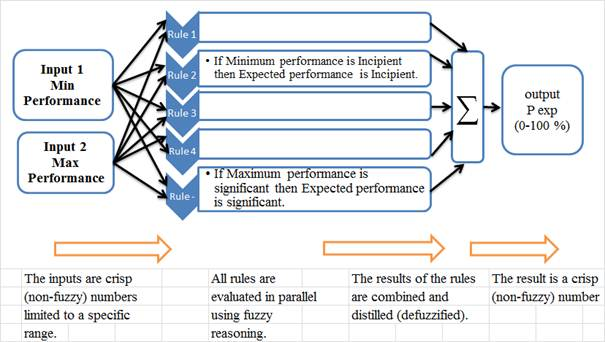Fig. 3. Expected Risk Performance model (Pexp).Fig. 4. Risk contingency model.

4.2.1. Step 1. Fuzzify Inputs

The first step is to take the inputs and determine the degree to which they belong to each of the appropriate fuzzy sets via membership functions. A membership function (MF) is a curve that defines how each point in the input space is mapped to a membership value (or degree of membership). The used bell membership function is specified by three parameters and has the function name gbellmf. Because of their smoothness and concise notation, bell membership functions are popular methods for specifying fuzzy sets. Its curves have the advantage of being smooth and nonzero at all points as displayed in figure 5. Before the rules can be evaluated, the inputs must be fuzzified according to each of these linguistic sets.

4.2.2. Step 2. Apply Fuzzy Operator

Once the inputs have been fuzzified, the degree to which each part of the antecedent has been satisfied for each rule. If the antecedent of a given rule has more than one part, the fuzzy operator is applied to obtain one number that represents the result of the antecedent for that rule. Figure 6 illustrate an example of the AND operator MIN. When evaluate the antecedent of the rules for the risk contingency, the two different pieces of the antecedent (company risk is incipient and project risk is significant). The fuzzy AND operator simply selects the minimum of the two values and the fuzzy operation for rules is complete.

4.2.3. Step 3. Apply Implication Method

A consequent is a fuzzy set represented by a membership function, which weights appropriately the linguistic characteristics that are attributed to it. The consequent is reshaped using a function associated with the antecedent (a single number). The input for the implication process is a single number given by the antecedent, and the output is a fuzzy set. Implication is implemented for each rule as displayed in figure 6.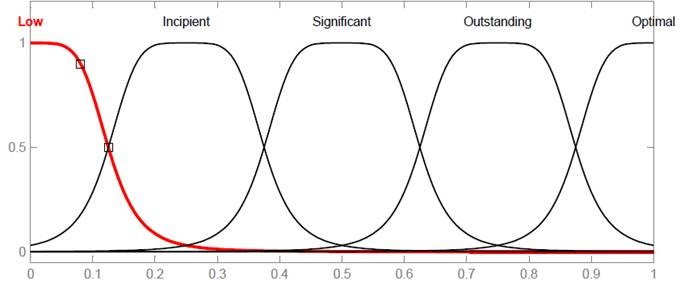Fig. 5. Member ship functions of input variables for risk contingency.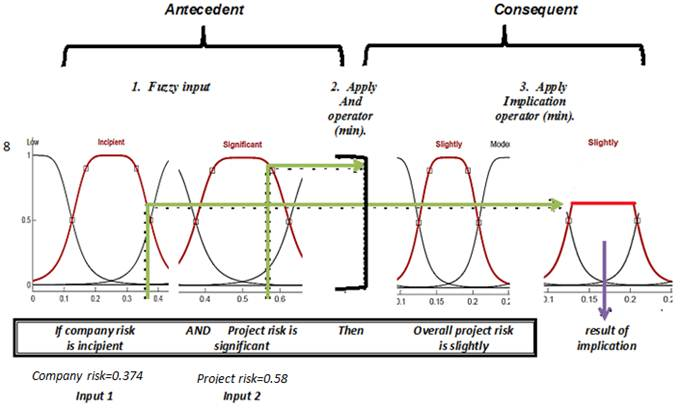Fig. 6. Implication Method Diagram.

4.2.4. Aggregate All Outputs

Aggregation is the process by which the fuzzy sets that represent the outputs of each rule are combined into a single fuzzy set. The output of the aggregation process is one fuzzy set for each output variable. In the diagram below, all rules have been placed together to show how the output of each rule is combined, or aggregated, into a single fuzzy set whose membership function assigns a weighting for every output (Contingency) value as appear in figure 7.

4.2.5. Step 5. Defuzzify Process

The input for the defuzzification process is a fuzzy set (the aggregate output fuzzy set) and the output is a single number. The most popular defuzzification method is the centroid calculation, which returns the centre of area under the curve. Figure 7 illustrate Defuzzifying process.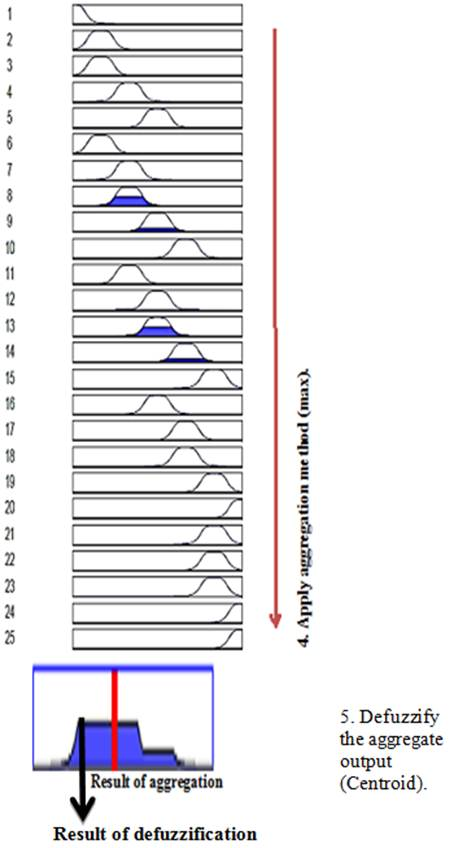Fig. 7. Aggregate All Outputs diagram.

4.3. Building System with Matlab Software

There are two types of fuzzy inference systems that can be implemented in the Fuzzy Logic Toolbox in MATLAB software: Mamdani-type and Sugeno-type. Mamdani’s fuzzy inference method is the most commonly seen fuzzy methodology. The next part describes in detail the steps which are followed to build the program based on fuzzy approach using MATLAB software.

4.3.1. Fuzzy Logic Toolbox

One of the primary goals of the Fuzzy Logic Tool box is to have an open and easily modified fuzzy inference system structure. The next section describes exactly building and implementing a fuzzy inference system using the tools provided for the first model to determine the expected performance of risk factors. [Source, Matlab fuzzy logic toolbox]

4.3.2. The FIS Editor

The FIS Editor handles the high-level issues for the numbers of inputs and outputs variables and their names. The FIS Editor displays general information about a fuzzy inference system.

4.3.3. The Membership Function Editor

Membership functions for fuzzy sets are defined, representing the performance levels for the input factors (P1, P2) and are used in processing the information.

1. The input Membership functions for point P1 which represents the minimum performance level of risk.

2. The input Membership functions for point P2 which represents the maximum performance level of risk.

3. The output Membership functions for point Pexp which represents the Expected performance level of risk.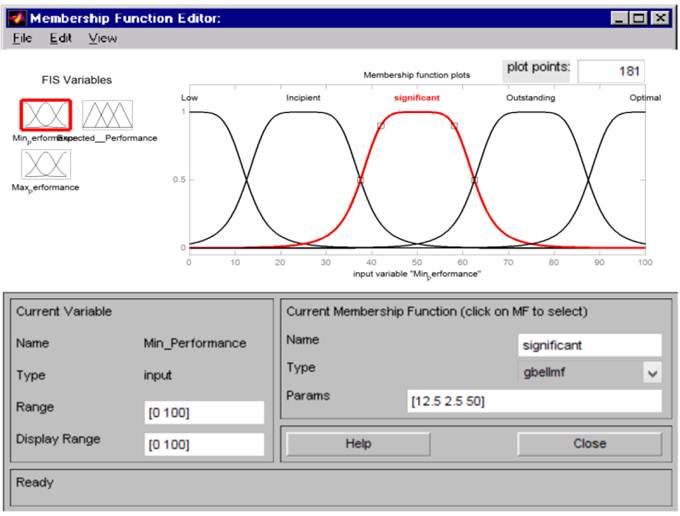Fig. 8. The Membership Function Editor for Input 1 (Minimum Performance point P1).

The performance value of the factors is given in the x-axis and the membership degree for each level of performance is given in the y-axis, where 1 is the total membership and 0. The non-membership. Figure 8 displays input Membership Function for point P1 and another input P2 and output Membership Function as the same membership function. Membership functions represented by bell function given by the equations (2).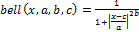Equation (2) (Carreño, 2004)  Where the parameter b is usually positive.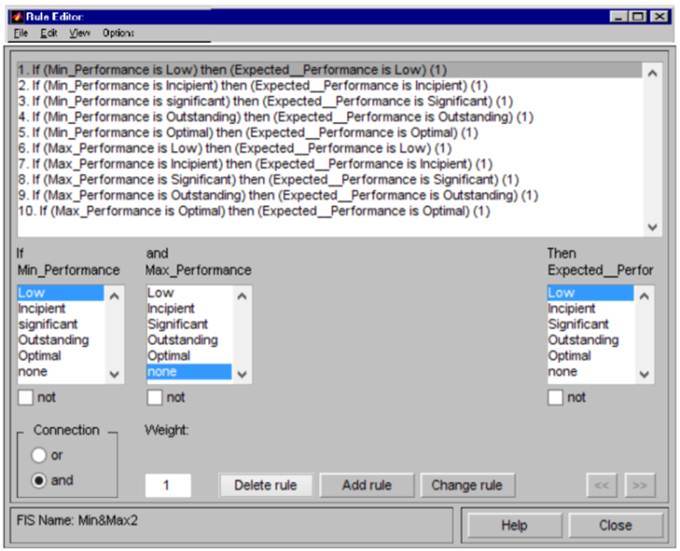Fig. 9. MATLAB Screen shoot of Rule editor.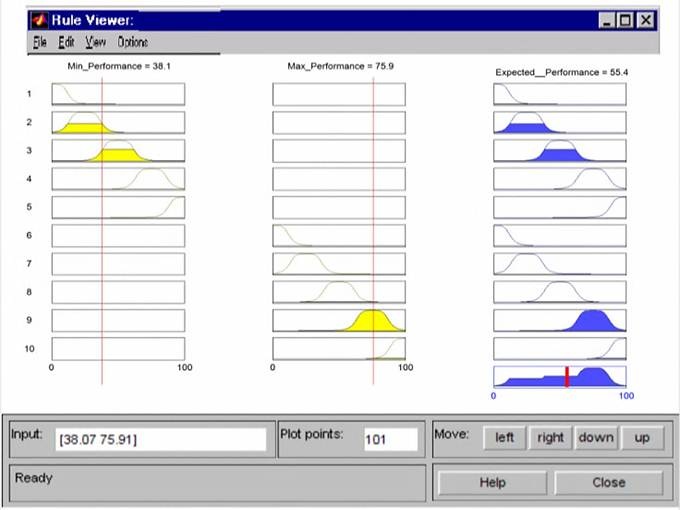Fig. 10. MATLAB Screen shoot of Rule Viewer.

4.3.4. The Rule Editor, the Rule Viewer and Surface Viewer

The Rule Editor represented with if... and then... rule, variables rule are considered as independent of each other so as to simplify the procedure (Fig 9). The rule viewer displays a roadmap of the whole fuzzy inference process. The figure displays 31 small plots as displayed in figure (10). The Surface Viewer presented a two-dimensional curve that represents the mapping from the rang of risk performance to Expected performance amount.

5. Effectiveness

Effectiveness for the value Obtained by the defuzzification of the linguistic values (PExpected) is the value obtained by sigmoidal function type, given parametrically by the equations 3 .

Figure 11 displays the Effectiveness degree of the risk performancevalue ccording to Carreno 2004 using sigmoidal function type.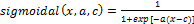Equation (3) Where ɑ: controls the slope at the crossing point, 0.5 of membership and equal 0.104, X is Performance at X axis and C =50.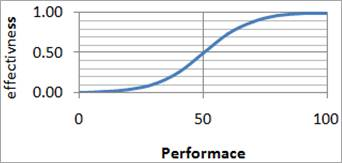Fig. 11. Effectiveness degree of the risk performance. (Carreño, 2004). 

The form and coverage of these membership functions follow a non-linear behaviour, in the form of a sigmoid, as proposed by Carreño et al (2004) in order to characterize performance, whose shape corresponds to the sigmoidal function shows in Figure 11, in which the effectiveness of the risk is represented as a function of the performance level. 

6. Model Verification

Five projects in different countries were selected to verify model application.

6.1. Part 1: AHP Survey

It was required form the participants to make pairwise comparison among risk factors and risk sub factors represent the relative importance between them based on the numerical scale (1-9) using Analytical Hierarchy Process (AHP) [19, 20, 21, 22, 23]

6.2. Part 2: Assigning Risk Performance

It was required form the participants to assign 3 point represent the low risk performance (P1), the high point of risk performance (P2) and the Expected risk performance (P Expected) for all sub factors in both company and project risk factors based on the numerical scale (1-9). 

6.3. Building Excel Spread Sheet Program

Excel spread sheet software was designed to solve the matrices using AHP concepts and Eigen value method to calculate risk factors weights.

The results obtained from fuzzy program represent (Expected Risk Performance PExpected) put in the Excel sheet (Column 23) in order to calculate risk effectiveness using sigmoidal function hence the overall risk can be determined upon equation no (1), figure 12 displays the Excel spread sheet with description for each column properties and function.

6.4. Model Results for Five Projects

The model applied on five projects in company level and project level through questionnaire as displayed in table (3) and the input data from questionnaire forms and output results of the program displayed in the following screen shoot of the excel program for project no (1) in Saudi Arabia for company level risk factors figure (12). The overall results of projects in both two levels displayed in table (3).

7. Risk Models Results Analysis

The projects holistic evaluations and the decomposed evaluation of the four (Diaz, P2 Only, P2=100 and fuzzy) approaches for each project profile provided in table 3.

Table 3. Company and project risk indexes and contingency value for each project conjunction with each.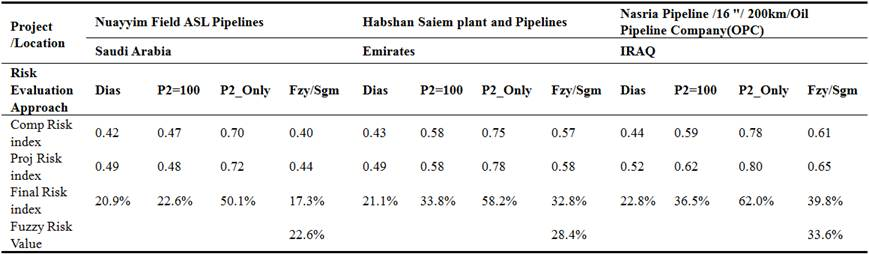Table 3. Continued.

 Project /Location Sareer Plant / Entisar field Pipeline 195 km Desouq Fields development pipelines - 132 Km Libya Egypt Risk Evaluation Approach Dias P2=100 P2_Only Fzy/Sgm Dias P2=100 P2_Only Fzy/Sgm Comp Risk index 0.36 0.39 0.64 0.36 0.31 0.28 0.55 0.19 Proj Risk index 0.35 0.34 0.53 0.32 0.46 0.37 0.64 0.27 Final Risk index 12.7% 13.4% 34.5% 11.7% 14.3% 10.5% 34.6% 5.2% Fuzzy Risk Value 19.8% 16.2%

Results of the project profiles decomposed evaluations for each study group were calculated for the four approaches and plotted against holistic evaluations for final project risk index; the results are shown in figure 16. The figure shows that in P2 Only and P2=100 approaches, most of decomposed evaluations were bigger than Diaz approach evaluations. This was because of the assumption that performance level point P1 was kept equal zero in these two approaches, so that any factors performance less than P1 and bigger than zero had a worth score value and will be included in the evaluation of the total value of the project (eq. 1) while in Diaz approach; the factors performance level point P1 was considered in the evaluations so that all the factors performance levels located behind P1 had zero worth score resulted in zero worth value and it will be excluded from the equation 1.

This figure also shows that ‘P2 Only approach had bigger values than P2 =100 approach, this was referred to that in P2 Only approach, the performance level points P2 provided by respondents were considered the extreme points of desirable performance and worth 100 points even it was not at the extreme end of the performance scale and all the attributes performance levels located after this point would have the same worth score. While in P2 100 approach the attributes performance point P2 was always kept at the end of the performance scale so that for attributes of performance point P bigger than the estimated P2 point by respondents. Their worth scores would be less than 100 point which of course, resulted in worth values less than that of P2 only approach.

The holistic evaluation curve was also included in this figure to compare the differences between the five approaches results and the holistic evaluations. figure16 shows that P2=100 approach curve and fuzzy approach are the closest one to each other, moreover they are the closest one to the holistic curve which means that they are the best approaches to capture the holistic approach.

Fuzzy approach model is more accurate than other models because it depends on fuzzy program to assess the minimum and maximum risk performance to evaluate the expected risk performance instead of using questionnaire in previous method, in addition the new model using nonlinear function to evaluate the risk factors effectiveness instead of linear functions in previous approaches.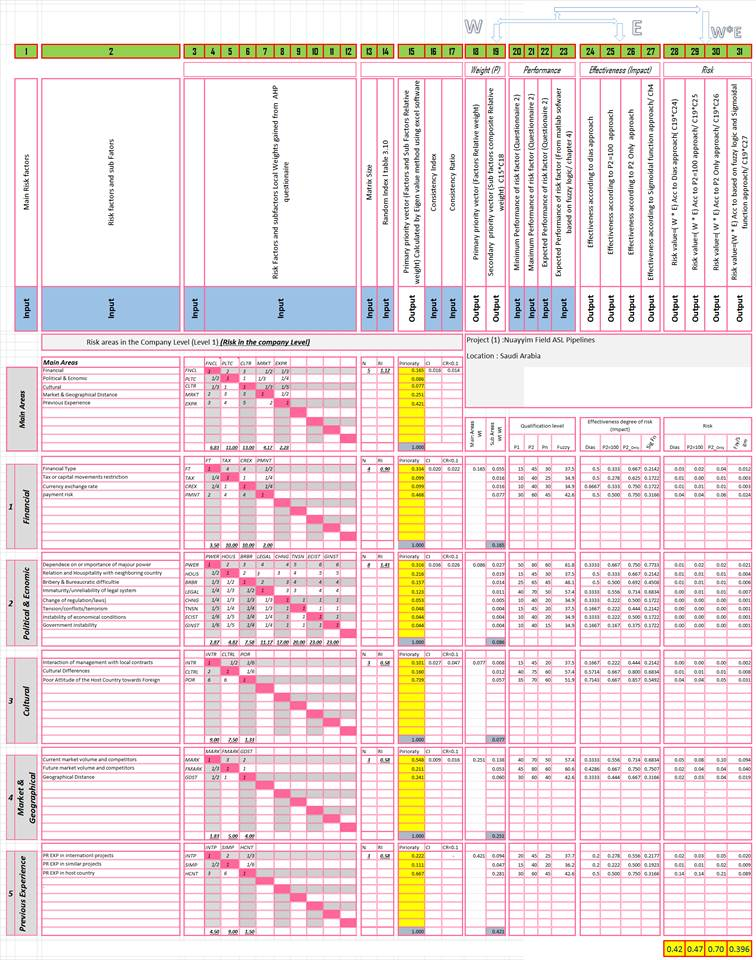Fig. 12. Screen shot for Excel sheet program explaining each columns identification and demonstrate the input data and output results of the program for risks in the company level in the project 1.Fig. 13. Overall Project risk index for decomposed approach for each project.

8. Model Validation

The purpose of the model validation process is to present statistics methods to validate the risk evaluation model results, hence using the validated results in evaluation the overall risk contingency using MATLAB software based in FUZZY logic approach.

Dias and Ionone, 1996 mentioned that the use of external criteria to objectively assess the validity of the evaluation models is a difficult issue because multi factors decision models are essentially subjective in nature. Therefore, past research has relied on indirect approaches, such as convergent validation, predictive validation, and axiomatic validation methods.

8.1. Holistic Evaluation

Holistic assessment (also called 'integrated assessment') focuses on the assessment of whole work activities rather than specific elements. Holistic evaluation is a direct evaluations made by the professional decision makers.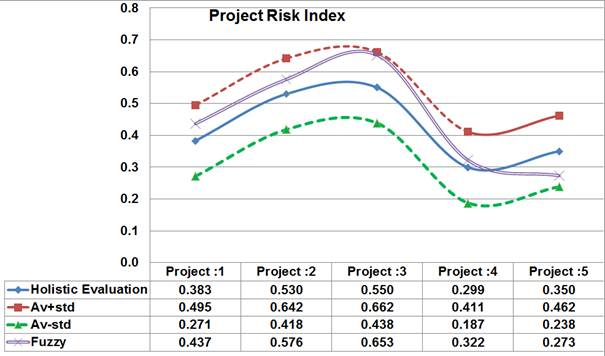Fig. 14. Convergent validation of developed fuzzy model results for project risks.

8.2. Convergent Validation

Convergent validation consists of comparing the results obtained by a fuzzy model with the holistic that is, direct evaluations made by the decision makers(average, average plus standard deviation, and average minus standard deviation values.

Figure (14) show the developed fuzzy model results, in addition to the holistic evaluation for company and project level risks. It is interesting to note that the developed fuzzy model results lies in the range of average plus standard deviation, and average minus standard deviation values.

8.3. Correlation Coefficient, R (Pearson Product Moment Correlation)

Correlation is a technique for investigating the relationship between two quantitative, continuous variables. The quantity r, called the linear correlation coefficient, measures the strength and the direction of a linear relationship between two variables. The linear correlation coefficient is sometimes referred to as the Pearson product moment correlation coefficient.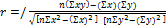Equation (4) [9, 17, 18] The correlation process was made by calculating the Pearson’s product- moment correlation coefficients between the holistic approach and the four decomposed approaches for each project profile for company and project levels results to verify the validity of fuzzy model and to determine which approach was the most closest to capture the holistic one. The results shown in table (4), indicate that the Pearson correlation coefficients in the four approaches resulted in that fuzzy approach was the one who mostly captures the holistic approach.

Table 4. Correlation Coefficient for each model results in addition to the holistic evaluation.

 Pearson Coefficient Model Holistic Diaz P2=100 P2 Only Fuzzy Company Level 1.00 092 0986 0.985 0.992 Project Level 1.00 0.78 098 0.94 0.95

8.4. Test Factor

Test factor validation was used to test the designated model and verify its robustness in predicting construction project’s risk. The model results are compared to this holistic evaluation using the test factor in model as follows:

Test Factor (TF) = RMR/RHE  Equation  (5), 

The test factors results of the holistic and decomposed models evaluations for the risks in the company and project levels are shown In table (5) show that fuzzy approach is the closest to the holistic that means; it is the closest approach to capture the Holistic. The previous test factor reveals that the accuracy and robustness of FUZZY model in company level have been tested using holistic evaluation, which proves its robustness in risk assessment (97%) in company level and (105%) in project level as appear in table 5.

Table 5. Test Factor for decomposed approach.

 Test Factor For models Risk assessment model Holistic Diaz P2=100 P2Only Fuzzy Company Level 100 % 96 % 109 % 167 % 97 % Project Level 100 % 113 % 114 % 168 % 105 %

8.5. Coefficient of Determination r 2

The coefficient of determination is a measure of how well the regression line represents the data. If the regression line passes exactly through every point on the scatter plot, it would be able to explain all of the variation. The further the line is away from the points, the less it is able to explain. The coefficient of determination, r2 gives the proportion of the variance (fluctuation) of one variable that is predictable from the other variable. It is a measure that allows us to determine how certain one can be in making predictions from a certain model/graph. The coefficient of determination, r2, is useful because it gives the proportion of the variance (fluctuation) of one variable that is predictable from the other variable. The correlation was made between holistic and decomposed evaluations for the four approaches for Company Risk model results. Figure (15, 16) displays the correlations between risk factors holistic and decomposed evaluations of the project profile for the four alternative approaches and their regression lines showing that the trend line of fuzzy approach is the closest one to the 45-dcgree line and the decomposed evaluations values in this approach are the closest to capture the holistic evaluations values (correlations for Diaz, P2=100, P2 Only, and fuzzy approaches are 0.850, 0.971, 0.971, 0.984 respectively) for Company Risk model results. (Correlations for Diaz, P2=100, P2 Only, and fuzzy approaches are 0.604, 0.952, 0.876, 0.899 respectively) for project risk model results.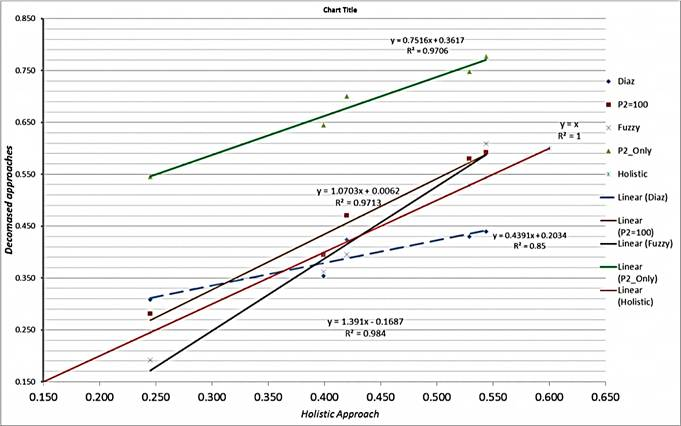Fig. 15. The correlations between risks attributes holistic and decomposed evaluations of the project profile for the four alternative approaches and their regression lines (company level risk).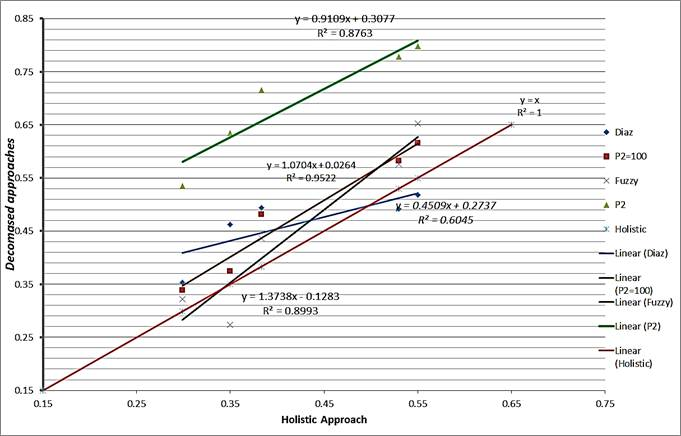Fig. 16. The correlations between risks attributes holistic and decomposed evaluations of the project profile for the four alternative approaches and their regression lines (company level risk).

9. Final Risk Value (Project Risk Contingency Value)

Cost overhead risk is obtained by aggregation and defuzzification of final company risk rating and project risk rating. Figures 17 displays Membership functions for input company and project risk index obtained from excel program concerning fuzzy approach, Figure 18 displays Membership functions for output final risk.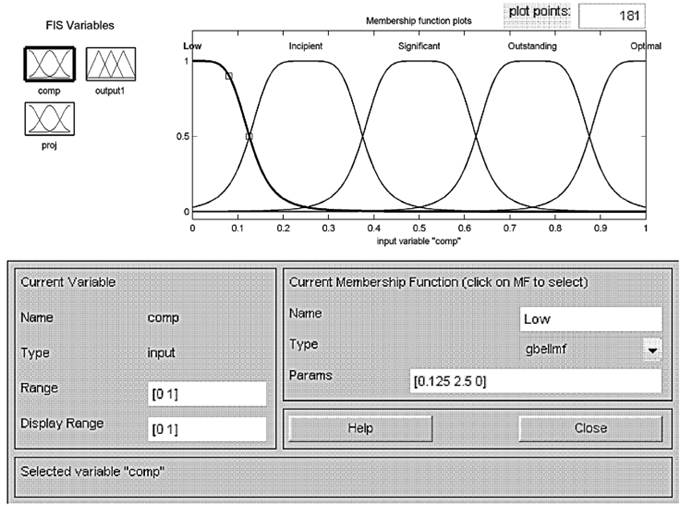Fig. 17. Screen shot of input Membership functions for company and project risk from Matlab program software.

Rules decision matrix are depicted in table (6) showing aggregation rules combining company risk with project risk to produce overall project risk value. These rules reflect the opinions of experts that participated to the brainstorming sessions and they may change with respect to risk attitude of different experts and different corporate policies which are company-specific and each company has its own risk knowledge leading to different fuzzy rules and may have different risk attitudes.

The philosophy of aggregated rules is closed to the risk priorities for water pipeline derived by (Cooper D., et al. . The important concern to determine the risk value the assumption if the project risk is very high and company risk is very high what would be the percentage of risk proportional to the overall budget.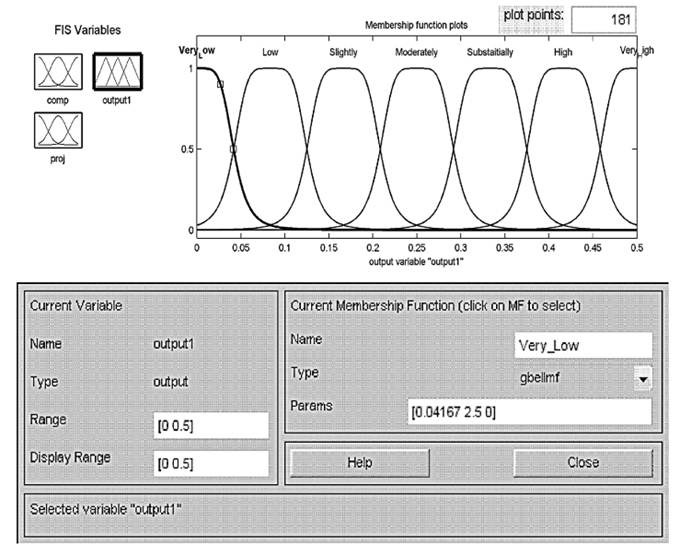Fig. 18. Screen shot of output Membership functions for final risk (Matlab program software).

Also this assumption reflect the opinions of decision makers and they may change with respect to risk attitude of different decision makers and different corporate policies which are company-specific and each company has its own risk knowledge leading to assumption and may have different risk attitudes.

Experts in this study assume that if the project risk is very high and company risk is very high, the percentage of risk proportional to the overall budget should be not less than %50 of the overall budget as shown in figure (20). For project (1) Nuayyim Field ASL Pipelines project (in Saudi Arabia), company risk is 0.40 and project risk 0.44 (based on fuzzy approach) The output of the fuzzy risk assessment procedure is a final risk cost, which is found to be 0.226 from the total budget as shown in (figure 20). Table (3) illustrated Fuzzy risk contingency value for each project based upon the program.

Table 6. Decision matrix showing aggregation rules combining company risk with project risk producing overall project risk value.

 Project Risk Low Incipient Significant Outstanding Optimal Company Risk Optimal High High High Extremely High Extremely High Outstanding moderately substantially substantially High Extremely High Significant Slightly moderately moderately substantially High Incipient Low Slightly Slightly moderately substantially Low V. L Low Low Slightly moderately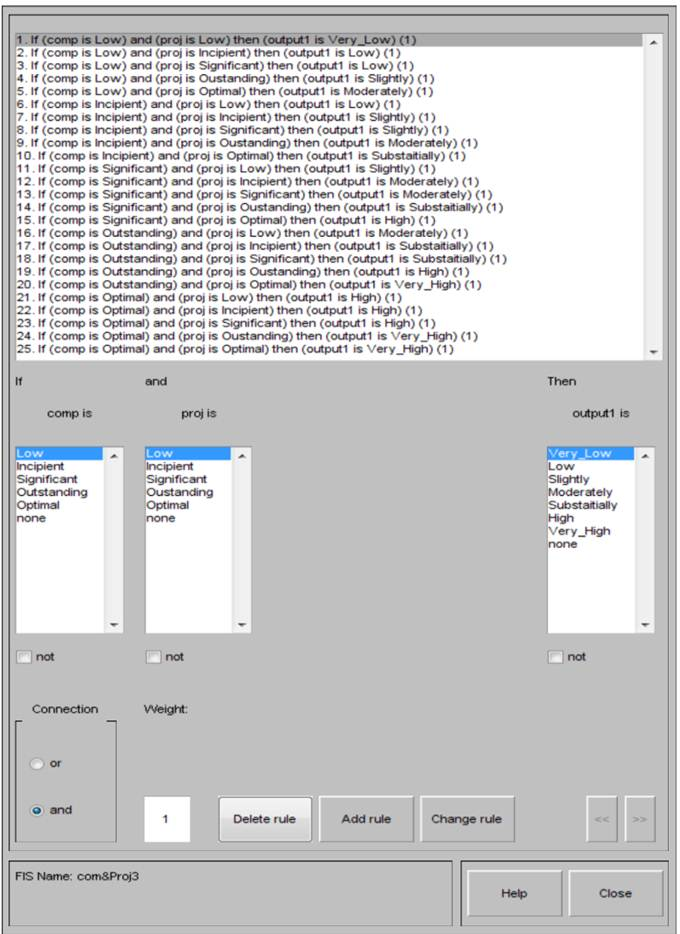Fig. 19. Aggregation rules combining company risk with project risk producing overall project risk value.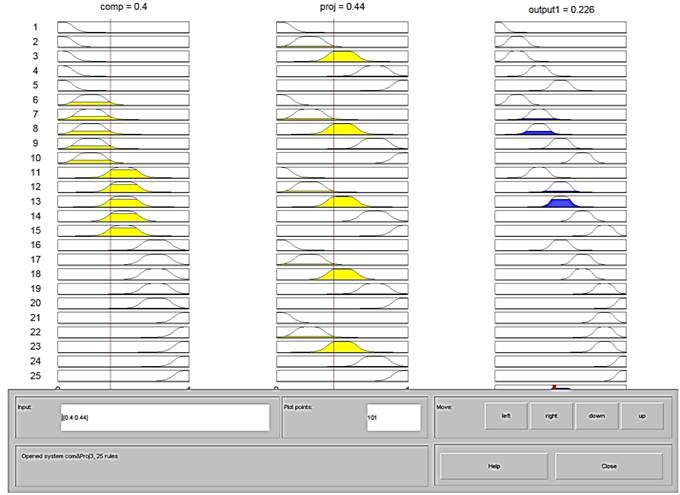Fig. 20. Aggregation and defuzzification process showing aggregation rules combining company risk with project risk producing overall project risk value.

10. Conclusion

The high-risk nature of international construction projects has led to many cost overruns in the history of construction. Therefore, contractors should use a systematic approach to manage risks on a project. The first step in managing risk is the identification and evaluation of risk sources. This study proposes a risk index (R) that performs three functions: evaluate sources of risk and uncertainty, accordingly prioritize international construction projects and evaluate project risk contingency value. A model for calculating the R-index was designed using the analytic hierarchy process (AHP) to evaluate risk factors weights (likelihood) and FUZZY LOGIC approach to evaluate risk factors impact (Risk Consequences) using software aids such as EXCEL and MATLAB software. Fuzzy risk assessment provides a promising tool to quantify risk ratings where the risk impacts are vague and defined by subjective judgments rather than objective data.

The model components were explained and discussed in detail throughout this paper. Applicability of the proposed methodology has been tested on real cases. Five case studies in different countries were selected to implement the designed models and test its results.

The developed model can be used to sort projects based upon risk, which facilitate company’s decision of which project can be pursued. The developed R model is tested, which prove its robustness in risk assessment (97%) in company level and (105%) in project level. It can also be used to sort construction projects based upon risk. It is an essential tool to assess the level of risk associated with construction projects under study in the bidding phase in order to take preventive actions.

The developed contingency risk model Demonstrate the ability to evaluate risk contingency value by aggregating rules combining company risk index and project risk index using fuzzy logic approach and MATLAB software.

Findings of the cases studies demonstrate that the proposed model can be easily applied to quantify risk ratings. The advantage of the tool is that it can provide guidance for the company about the amount of risk premium that should be included in the mark-up.

This model proved that fuzzy logic approach, which uses experts’ knowledge, overcomes lack of data and the uncertainty in forecasting future events. It is anticipated that this model could provide a very wide range of use in estimating whole life costs of public service.

Acknowledgements

The authors express their gratitude to Pr. Hesham Abdel Khalek and Dr. Remon Aziz for their generous contribution of time, energy, and knowledge in this study. They also extend their gratitude to all companies that facilitate the authors’ research and participate in this research.

References

1. Antonio J., Monroy A., Gema S. and Angel L., (2011). "Financial Risks in Construction Projects". African Journal Of Business Management Vol. 5(31), Pp. 12325-12328,7 December, 2011.
2. Carreño1, M. L., Cardona, O. D. and Barbat, A. H. (2004). "Evaluation of the Risk Management Performance". 250th Anniversary Of The 1755 Lisbon Earthquake, 1technical University Of Catalonia, Barcelona, Spain.
3. Deng X. And Low. (2012). "Understanding The Critical Variables Affecting The Level Of Political Risks In International Construction Projects". KSCE Journal of Civil Engineering (2013) 17(5):895-907.
4. Dias A, Ioannou P.(1996). "Company and Project Evaluation Model for Privately Promoted Infrastructure Projects. Journal of Construction Engineering and Management, ASCE 1996; 122(1):71–82. March.
5. Dikmen I, Birgonul T and Han S. (2007). "Using Fuzzy Risk Assessment to Rate Cost Overrun Risk in International Construction Projects". International Journal of Project Management 25 (2007) 494–505.
6. Enrique J., Ricardo C., Vicent E. and Jerónimo A.,(2011), Analytical Hierarchical Process (AHP) As A Decision Support Tool In Water Resources Management, Journal Of Water Supply: Research And Technology—Aqua | 60.6 | 2011.
7. Garshasb A., Mostafa A. and Abas A., (2012). "Fuzzy Adaptive Decision Making Model for Selection Balanced Risk Allocation". International Journal of Project Management 30 (2012) 511–522.
8. Hyun- C., Hyo- C. And. Seo j., (2004). "Risk Assessment Methodology for Underground Construction Projects", ASCE Journal of Construction Engineering and Management, 130, 258-272.
9. Jessica M., (2014), "Pearson Correlation Coefficient: Formula, Example & Significance". Http://Education- Portal.Com/Academy /Lesson/Pearson-Correlation-Coefficient-Formula-Example-Significance.Html#Lesson.
10. John G. Edward G.(2003). "International Project Risk Assessment: Methods, Procedures, and Critical Factors". A Report Of The Center Construction Industry Studies The University Of Texas At Austin.
11. Liu Jun A, Wang Qiuzhen B, Ma Qingguo B.(2011). The Effects of Project Uncertainty and Risk Management on IS Development Project Performance". A Vendor Perspective International Journal of Project Management 29 (2011) 923–933.
12. Lotfi A. Zadeh. (2002). "Fuzzy Logic Toolbox for Use with MATLAB, User’s Guide".
13. Ludovic V, Marle F, Bocquet J, C. (2011). "Measuring Project Complexity Using the Analytic Hierarchy Process". International Journal of Project Management 29 (2011) 718–727.
14. Mag Malek. (2000). "An Application of Fuzzy Modeling in Construction Engineering". International Proceedings of the 36th Annual Conference of the Associated Schools of Construction (ASC), 287-300.
15. Ming W., And Hui Ch. (2003). "Risk Allocation and Risk Handling of Highway Projects In Taiwan". Journal of Management in Engineering, Asce / April 2003.
16. Prasanta D. (2002). "An Integrated Assessment Model For Cross Country Pipelines". Environmental Impact Assessment Review, 22, (2002) 703–721.
17. Pearson Correlation Coefficient Calculator.(2014), Http://Www. Socscistatistics. Com/Tests /Pearson /Default2.Aspx.
18. Pearson's Correlation Coefficient, Data Analysis, 2014, Http:// Learntech.Uwe.Ac.Uk/Da/ Default. Aspx? Pageid=1442.
19. Prasanta D.(2010). "Managing Project Risk Using Combined Analytic Hierarchy Process and Risk Map". Applied Soft Computing 10 (2010) 990–1000.
20. Saaty TL. The Analytic Hierarchy Process. 1980. New York: Mc graw- Hill, 1980.
21. Saaty TL. Decision Making For Leaders. Belmont, California: Life Time Leaning Publications, 1985.
22. Saaty TL. (1990). "How to Make a Decision: The Analytic Hierarchy Process". European Journal of Operational Research, North-Holland 1990; 48:9±26.
23. Saaty TL, Kearns KP.(1991). "Analytical Planning: The Organization of Systems". The Analytic Hierarchy Process Series 1991; Vol. 4RWS.
24. Salman A. (2003). "Study Of Applying Build Operate And Transfer Bot Contractual System On Infrastructure Projects In Egypt". PHD Thesis, Zagazig University, Faculty of Eng.
25. Sou L., AnC., And Chung Y. (2001). "A GA_ based Fuzzy Optimal Model For Construction Time-Cost Trade Off". International Journal of Project Management, 19(1), 47-58.
26. Wang, N., Horner and M. El-Haram. (2004). "Fuzzy Logic Approach To A Generic Elemental Whole Life Costing Model", Twentieth Annual Conference Of Association Of Researchers In Construction Management, Vol. 1, 383-391, Edinburgh.
27. Zayed T, Mohamed A, Jiayin P.(2008). "Assessing Risk And Uncertainty Inherent In Chinese Highway Projects Using AHP "Internal Journal Of Project Management" 26 (2008) 408–419.
28. Bu-Qammaz, A.S.(2007), "risk assessment of international construction projects using the analytic network process", master of science thesis, middle east technical university.
29. Cooper D, Grey S, Raymond G and Walker P, 2007, "Project Risk Management Guidelines: Managing Risk in Large Projects and Complex Procurements", John Wiley & Sons, Ltd, ISBN 0-470-02281-7.
30. Zayed, T, and Chang, L. (2002). "Prototype Model for Build-Operate-Transfer Risk Assessment. Journal of Management in Engineering / January 2002 / 7.

 Contents 1. 2. 3. 4. 4.1. 4.2. 4.3. 5. 6. 6.1. 6.2. 6.3. 6.4. 7. 8. 8.1. 8.2. 8.3. 8.4. 8.5. 9. 10.
Article ToolsAbstractPDF(9632K)